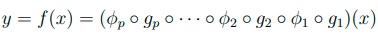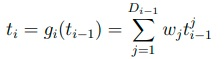Two key components in a neural network are neurons and connections between neurons. Each neuron or node in the neural network performs a function on the input and optionally uses a nonlinear activation function before outputting. A connection transfers the weighted output of one neuron to another neuron as input. Neurons are typically grouped as layers. Each layer is responsible for certain functionality such as convolution or normalization. Here, the multilayer perceptron is described for illustration.

A multilayer perceptron is one of the early networks. A multilayer perceptron with input x ∈ Rm and output y ∈ Rn can be treated as a function f : Rm → Rn . Assuming the multilayer perceptron has p layers excluding the input layer, the network is defined.where g1…p are affine functions or linear functions of input with a bias term and φ1…p are activation functions. The functions g1…p can be expressed as:where tji denotes the jth element of the output of the ith layer and Di is the dimension of ti . wj is the weight vector of length Di.

Several observations about the structure of the network can be made. First, the network is fully connected, meaning there is a connection between every two neurons from adjacent layers. This is not a necessary property for neural networks. Fully connected layers are computationally expensive if the dimension of input is high.

Second, functions gi are identical for all layers (excluding the input layer). This is typically not true for more advanced networks.

Three examples of activation functions are listed below:Activation functions are neither linear nor affine. This is significant because the tasks are almost certainly not linear. Another way to see the importance is that if the activation function is affine, all layers will collapse to one. In other words, the composition of affine functions is also affine.